# 998. 最大二叉树 II

## 问题

• 如果 $$A$$ 为空，返回 $$null$$
• 否则，令 $$A[i]$$ 作为 $$A$$ 的最大元素。创建一个值为 $$A[i]$$ 的根节点 $$root$$
• $$root$$ 的左子树将被构建为 $$Construct([A, A, ..., A[i-1]])$$
• $$root$$ 的右子树将被构建为 $$Construct([A[i+1], A[i+2], ..., A[A.length - 1]])$$
• 返回 $$root$$
请注意，我们没有直接给定 $$A$$，只有一个根节点 $$root = Construct(A)$$.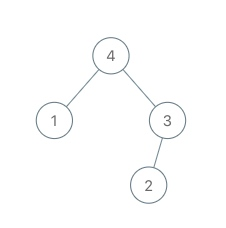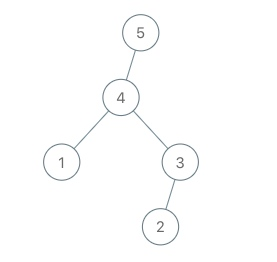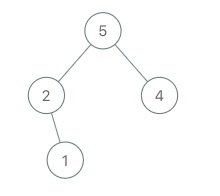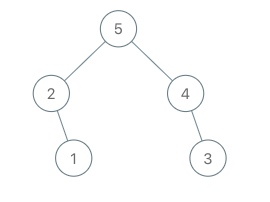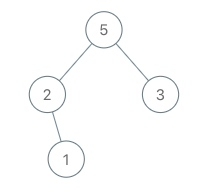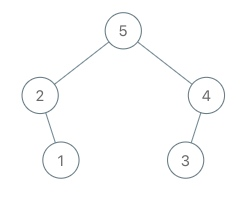## 解法

1. $$root$$为空，此时直接生成一个TreeNode(val)返回即可
2. $$root.val < val$$，此时说明，$$val$$已经找到了应该在的位置,那么生成一个TreeNode(val), 并且将左儿子设置成$$root$$（因为$$root$$对应的数组元素必然在$$val$$的左边。）
3. $$root.val > val$$,此时说明，$$val$$仍然没有找到所在位置，但是因为$$val$$在最右，那么递归到$$root.right$$中去处理。

## 代码

### java

/**
* Definition for a binary tree node.
* public class TreeNode {
*     int val;
*     TreeNode left;
*     TreeNode right;
*     TreeNode(int x) { val = x; }
* }
*/
class Solution {
public TreeNode insertIntoMaxTree(TreeNode root, int val) {
// 情况1
if (root == null) {
return new TreeNode(val);
}
// 情况2
if (root.val < val) {
TreeNode node = new TreeNode(val);
node.left = root;
return node;
}
// 情况3
root.right = insertIntoMaxTree(root.right,val);
//最终递归返回
return root;
}
}